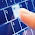## Pages

### Example:

----------------------------------
```#include "opencv2/core/core.hpp"
#include "opencv2/highgui/highgui.hpp"
#include "opencv2/imgproc/imgproc.hpp"
#include "iostream"

using namespace cv;
using namespace std;

int main( )
{

Mat src1;
namedWindow( "Original image", CV_WINDOW_AUTOSIZE );
imshow( "Original image", src1 );

Mat gray;
cvtColor(src1, gray, CV_BGR2GRAY);
namedWindow( "Result window", CV_WINDOW_AUTOSIZE );
imshow( "Result window", gray );

// Use the copy constructor
Mat src2(src1);
namedWindow( "window2", CV_WINDOW_AUTOSIZE );
imshow( "window2", src2 );

// Assignment operator
Mat src3;
src3 = src2;
namedWindow( "window3", CV_WINDOW_AUTOSIZE );
imshow( "window3", src3 );

// Selecting a region of interest using a rectangle
Mat src4 (src1, Rect(50, 50, 150, 150) );
namedWindow( "window4", CV_WINDOW_AUTOSIZE );
imshow( "window4", src4 );
// Another way of doing same
Rect r(10, 10, 100, 100);
Mat src41 = src1(r);
namedWindow( "window4.1", CV_WINDOW_AUTOSIZE );
imshow( "window4.1", src41 );

// clone() function
Mat src5 = src1.clone();
namedWindow( "window5", CV_WINDOW_AUTOSIZE );
imshow( "window5", src5 );

// copyTo() function
Mat src6;
src1.copyTo(src6);
namedWindow( "window6", CV_WINDOW_AUTOSIZE );
imshow( "window6", src6 );

// Using Range()
Mat P;
src6.rowRange(1,2).copyTo(P);
cout << "P = " << endl << " " << P(Range::all(),Range::all()) << endl << endl ;

// Range::all() - “the whole sequence”
// Range(a,b) - "[start , end]
// Copy {row : 0 to 4} and {col : 5 to 9} to matrix Q
Mat Q; Q = gray(Range(0,5),Range(5,10));
cout<< Q << endl << endl;

//make a black image from an existing image
src1 = Scalar(0);
namedWindow( "window7", CV_WINDOW_AUTOSIZE );
imshow( "window7", src1 );

Mat mtx(img); // convert IplImage* -> Mat
namedWindow( "window8", CV_WINDOW_AUTOSIZE );
imshow( "window8", mtx );

// Mat() Constructor
Mat M(2,2, CV_8UC3, Scalar(0,0,255));
cout << "M = " << endl << " " << M << endl << endl;

// Use C\C++ arrays and initialize via constructor
// Create a matrix with more than two dimensions
int sz = {4,2,3};
Mat L(3,sz, CV_8UC(1), Scalar::all(0));

// Create() function
Mat N;
N.create(4,4, CV_8UC(2));
cout << "N = "<< endl << " " << N << endl << endl;

// initializer: zeros(), ones(), :eyes(). Specify size and data type to use
Mat E = Mat::eye(4, 4, CV_64F);
cout << "E = " << endl << " " << E << endl << endl;

Mat O = Mat::ones(2, 2, CV_32F);
cout << "O = " << endl << " " << O << endl << endl;

Mat Z = Mat::zeros(3,3, CV_8UC1);
cout << "Z = " << endl << " " << Z << endl << endl;

// For small matrices you may use comma separated initializers:
Mat C = (Mat_<double>(3,3) << 0, -1, 0, -1, 5, -1, 0, -1, 0);
cout << "C = " << endl << " " << C << endl << endl;

Mat RowClone = C.row(1).clone();
cout << "RowClone = " << endl << " " << RowClone << endl << endl;

// Fill out a matrix with random values using the randu() function.
// You need to give the lower and upper value for the random values:
Mat R = Mat(3, 2, CV_8UC3);
randu(R, Scalar::all(0), Scalar::all(255));
cout << "R = " << endl << " " << R << endl << endl;

// allocates a 30x40 floating-point matrix
Mat A(30, 40, DataType<float>::type);

Mat B = Mat_<std::complex<double> >(3, 3);
// the statement below will print 6, 2 /*, that is depth == CV_64F, channels == 2 */
cout << B.depth() << ", " << B.channels() << endl;

waitKey(0);
return 0;
}```
----------------------------------

1.2.3.4.don't follow this fucking site otherwise you probably forget all about opencv

5.6.7.Best R Programming Training in Bangalore offered by myTectra. India's No.1 R Programming Training Institute. Classroom, Online and Corporate training in R Programming
r programming training

8.Very useful details provided by you. I hope it would be useful for many of the seekers. Thank you for sharing details here with us.
Home tutors are provided by TheTuitionTeacher in Delhi.
Home Tutors in Delhi | Home Tutors Delhi

9.IOT Training in Bangalore - Live Online & Classroom
Students are made to understand the type of input devices and communications among the devices in a wireless media.
IOT Training course observes iot as the platform for networking of different devices on the internet and their inter related communication.

10.I like your blog, I read this blog please update more content on python, further check it once at python online training

11.very informative blog and useful article thank you for sharing with us , keep posting learn more AngularJS Online Training Hyderabad

12.Currently Python is the most popular Language in IT. Python adopted as a language of choice for almost all the domain in IT including Web Development, Cloud Computing (AWS, OpenStack, VMware, Google Cloud, etc.. ),Read More

13.14.Our write my nursing research paper services are focused on making your life in school smooth and enjoyable in comparison to what you might be going through at the moment.

15.You need not to worry any more,we are here to offer you the best academic writing
solutions;order quality pre written research papers for sale from us today!

16.Thanks for Sharing This Article.It is very so much valuable content. I hope these Commenting lists will help to my website
top angular js online training

17.It is very good blog with full information, we are looking forward to connect with you in future. Best home tutors are provided by TheTuitionTeacher in Delhi and Lucknow.
Home Tutors in Delhi | Home Tuition Services

18.Hey Nice Blog!! Thanks For Sharing!!! Wonderful blog & good post. It is really very helpful to me, waiting for a more new post. Keep Blogging!Here is the best angularjs training online with free Bundle videos .

contact No :- 9885022027.

19.Here is the link of best BSc Cardiac Care Technology Colleges In Bangalore. If you need to get the list of best colleges, just click on the following link.
BSc Cardiac Care Technology Colleges In Bangalore

20.I am a regular reader of your blog and I find it really informative. Hope more Articles From You.Best
Tableau tutorial video available Here. hope more articles from you.